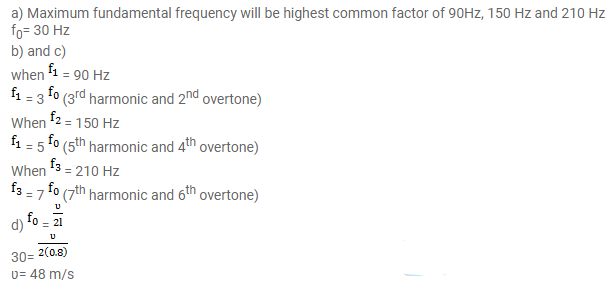# Three resonant frequencies of a string areQuestion:

Three resonant frequencies of a string are $90,150,210 \mathrm{~Hz}$.

a. Find the highest possible fundamental frequency of vibration of this string.

b. Which harmonics of the fundamental are the given frequencies?

c. Which overtones are these frequencies

d. If the length of the string is $80 \mathrm{~cm}$, what would be the speed of the transverse wave on this string?

Solution: